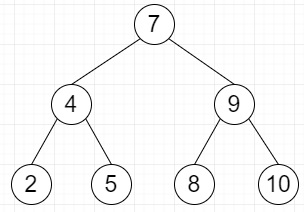# Find median of BST in O(n) time and O(1) space in Python

Suppose we have Binary Search Tree(BST), we have to find median of it. We know for even number of nodes, median = ((n/2th node + (n+1)/2th node) /2 For odd number of nodes, median = (n+1)/2th node.

So, if the input is likethen the output will be 7

To solve this, we will follow these steps −

• if root is same as None, then

• return 0

• node_count := number of nodes in the tree

• count_curr := 0

• current := root

• while current is not null, do

• if current.left null, then

• count_curr := count_curr + 1

• if node_count mod 2 is not 0 and count_curr is same as(node_count + 1) /2, then

• return previous.data

• otherwise when node_count mod 2 is 0 and count_curr is same as(node_count/2) +1, then

• return(previous.data + current.data) /2

• previous := current

• current := current.right

• otherwise,

• previous := current.left

• while previous.right is not null and previous.right is not same as current, do

• previous := previous.right

• if previous.right is null, then

• previous.right := current

• current := current.left

• otherwise,

• previous.right := None

• previous := previous

• count_curr := count_curr + 1

• if node_count mod 2 is not 0 and count_curr is same as(node_count + 1) / 2, then

• return current.data

• otherwise when node_count mod 2 is 0 and count_curr is same as(node_count / 2) + 1, then

• return(previous.data+current.data) /2

• previous := current

• current := current.right

## Example

Let us see the following implementation to get better understanding −

Live Demo

class TreeNode:
def __init__(self, data):
self.data = data
self.left = None
self.right = None
def number_of_nodes(root):
node_count = 0
if (root == None):
return node_count
current = root
while (current != None):
if (current.left == None):
node_count+=1
current = current.right
else:
previous = current.left
while (previous.right != None and previous.right != current):
previous = previous.right
if(previous.right == None):
previous.right = current
current = current.left
else:
previous.right = None
node_count += 1
current = current.right
return node_count
def calculate_median(root):
if (root == None):
return 0
node_count = number_of_nodes(root)
count_curr = 0
current = root
while (current != None):
if (current.left == None):
count_curr += 1
if (node_count % 2 != 0 and count_curr == (node_count + 1)//2):
return previous.data
elif (node_count % 2 == 0 and count_curr == (node_count//2)+1):
return (previous.data + current.data)//2
previous = current
current = current.right
else:
previous = current.left
while (previous.right != None and previous.right != current):
previous = previous.right
if (previous.right == None):
previous.right = current
current = current.left
else:
previous.right = None
previous = previous
count_curr+= 1
if (node_count % 2 != 0 and count_curr == (node_count + 1) // 2 ):
return current.data
elif (node_count%2 == 0 and count_curr == (node_count // 2) + 1):
return (previous.data+current.data)//2
previous = current
current = current.right
root = TreeNode(7)
root.left = TreeNode(4)
root.right = TreeNode(9)
root.left.left = TreeNode(2)
root.left.right = TreeNode(5)
root.right.left = TreeNode(8)
root.right.right = TreeNode(10)
print(calculate_median(root))

## Input

root = TreeNode(7)
root.left = TreeNode(4)
root.right = TreeNode(9)
root.left.left = TreeNode(2)
root.left.right = TreeNode(5)
root.right.left = TreeNode(8)
root.right.right = TreeNode(10)

## Output

7